### Home > A2C > Chapter 2 > Lesson 2.1.3 > Problem2-37

2-37.
1. Look back at the data given in problem 2-20 that describes the rebound ratio for an approved tennis ball. Suppose you drop a tennis ball from an initial height of 10 feet. Homework Help ✎

1. How high would it rebound after the first bounce?

2. How high would it rebound after the 12th bounce?

3. How high would it rebound after the nth bounce?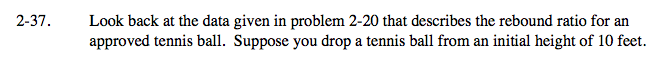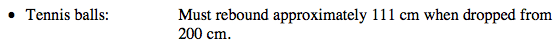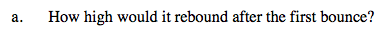Find the rebound ratio by dividing the rebound by the initial height.

$\frac{111}{200}=0.555$

Multiply the new initial height by the ratio.

10 · 0.555

5.55 ft.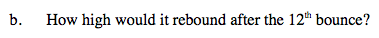Multiply the initial height by 0.555, twelve times.

10 · (0.555)12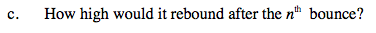Remember that the initial height is 10 feet and the ratio is 0.555.